• 点乘的公式：a*b= |a| * |b| * cosθ 由点乘的公式可知，点乘的结果不仅仅是由相似度（也就是cosθ）决定，还由向量的大小决定的，如果很大，即使向量夹角很大（也就是很不相似），得出来的结果也有可能很大...机器学习 深度学习
• 第1节：零向量 1.零向量的概念 　对于任意向量x，都有x+y=x，则x被称为零向量。例如，3D零向量为[0 0 0]。零向量非常特殊，因为它是唯一大小为零的向量，并且唯一一个没有方向的向量。 第2节：负向量 1.负向量的...

第1节：零向量

1.零向量的概念

对于任意向量x，都有x+y=x，则x被称为零向量。例如，3D零向量为[0 0 0]。零向量非常特殊，因为它是唯一大小为零的向量，并且唯一一个没有方向的向量。

第2节：负向量

1.负向量的概念

对于向量x，如果x+(-x)=0，则-x就是负向量。

2.负向量的运算法则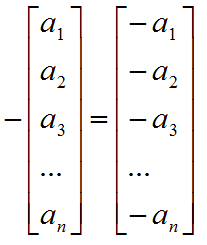将此法则应用到2D，3D，4D中，则

-[x y] = [-x -y]

-[x y z] = [-x -y -z]

-[w x y z] = [-w -x -y -z]

3.负向量的几何解释

向量为负表示将得到一个和原向量大小相等，方向相反的向量。

第3节：向量的模

1.向量的模的概念

所谓的向量的模就是指向量的大小或者说长度。

2.向量的模的运算法则

在线性代数中，向量的模通常用在向量两边各加两条竖线的方式表示，如||v||，表示向量v的模。向量的模的计算公式如下：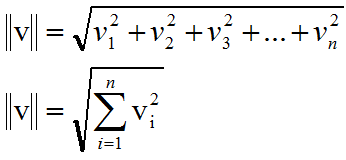对于2D，3D向量的如下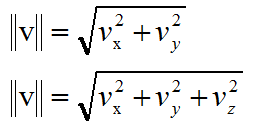第4节：标量与向量的运算

1.运算法则

虽然标量与向量不能相加减，但是可以相乘，至于标量与向量的除法可以看做乘以倒数。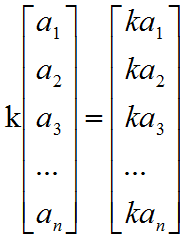对于2D，3D向量的如下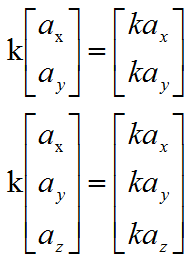2.几何解释

向量乘以标量或者除以标量，相当于以因子k来缩放向量的长度。

第5节：标准化向量

1.标准化向量的概念

所谓的标准化向量就是单位向量，就是向量的长度为1的向量。有时候也称作为法线。

2.运算法则

对于任意非零向量v，都能计算出一个和v方向相同的单位向量n，这个过程被称作为向量的“标准化”，要标准化向量，将向量除以它的大小（模）即可。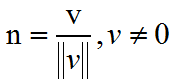第6节：向量的加法和减法

1.向量的加法和减法的前提

如果两个向量的维数相同，那么他们能够相加减，运算结果的向量的维数和原向量相同。

2.运算法则

向量的加法等于两个向量的分量相加，向量的减法相当于加上一个负向量。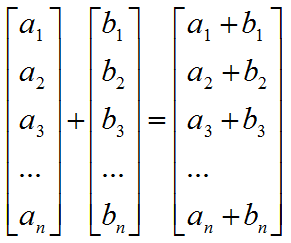3.几何解释

向量的加法和减法引导出了三角形法则，即将向量的首尾相连就会得到加法的结果，如下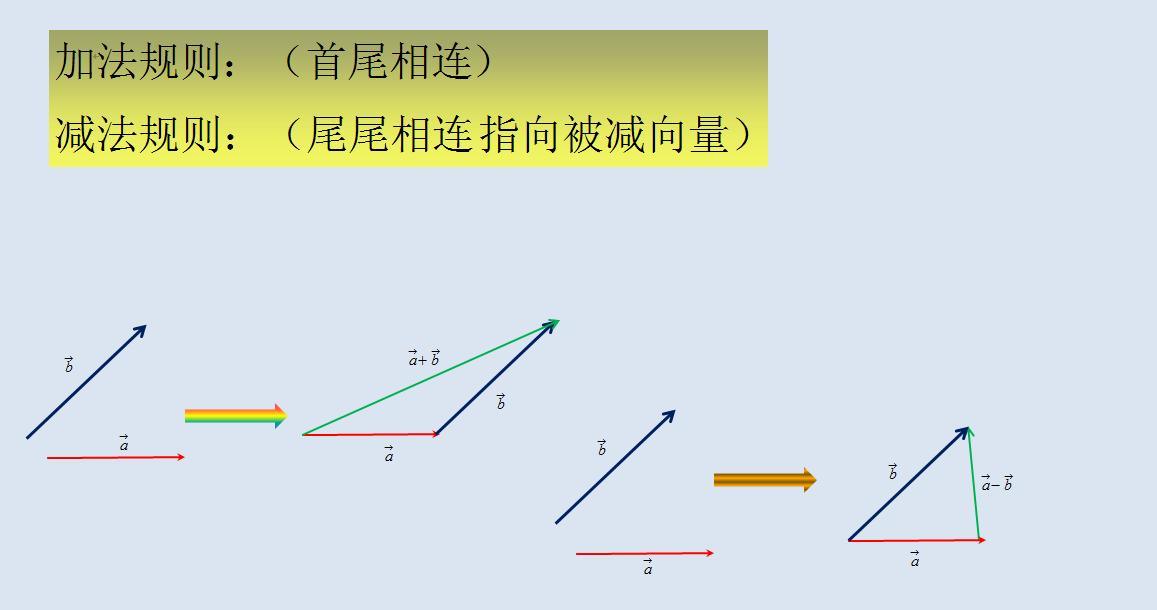第7节：距离公式

1.距离公式的推导

通过上面的三角形原则，我们可以发现，通过两个向量的加减可以得到第三个向量，我们将这个过程逆置，如果知道了两点的距离，如何求出其距离，我们可以利用向量的减法实现。

2.运算公式

在3D中，已知两点a，b，求两点之间的距离d？我们可以将a，b两点看做向量，然后b-a就是向量d，然后我们再计算向量d的模就是两点间的距离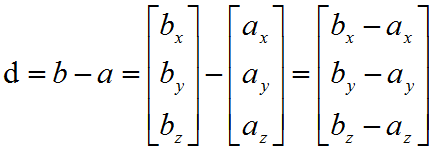求出向量d后，再求d的模就是两点的距离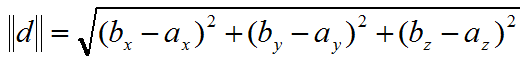第8节：向量的点乘

1.基本概念

标量可以和向量相乘，向量也可以和向量向量相乘，这就叫点乘，也叫做内积。标量与向量相乘不可以写点，向量与向量相乘必须要写点，向量的点乘优先级高于向量的加减法。注意：向量点乘后的结果是标量

2.运算法则

注意：向量点乘后的结果是标量，不再是向量。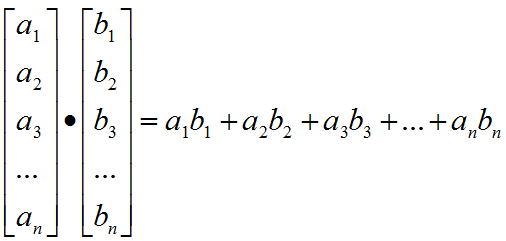应用到2D，3D中为

a·b = axbx + ayby

a·b = axbx + ayby+ azbz

3.几何解释

向量的点乘描述的是两个向量的相似程度，即两个向量之间的夹角的大小

向量的点乘的集合运算法如下，向量的点乘结果与cos函数有关，当两个向量垂直时，向量的点乘结果为0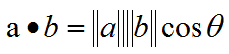第9节：向量的投影

1.基本概念

给定两个向量v和n，能将v分解成两个分量，一个是垂直于向量n，一个平行于向量n，平行于向量n的向量我们称为在向量n上的投影。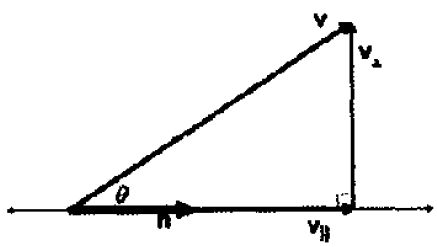2.投影的求解

因为向量n平行于投影向量，所以可以求出向量n的单位向量再乘以投影的模，就可以得到投影向量，如下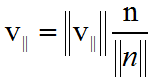我们接下来求投影的模即可，我们可以根据三角函数的余弦公式来求出投影的模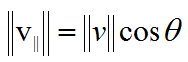代入投影的模就可以求出投影向量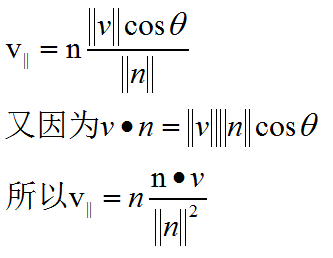3.垂直向量的求解

根据三角形法则，可以轻易求出垂直的向量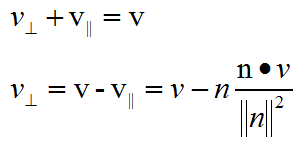第10节：向量的叉乘

1.基本概念

两个向量的叉乘得到是向量，且这个向量垂直于原来的两个向量。向量的叉乘只可以运用在3D向量中。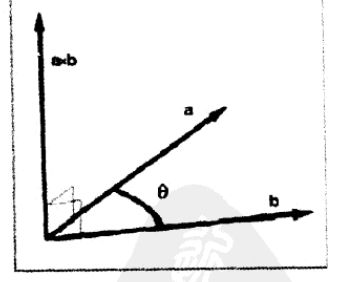2.数学运算公式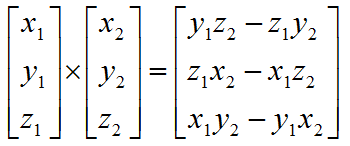3.几何运算公式

向量叉乘的结果向量的长度与两个向量的夹角有关，且成正弦函数关系，如果向量a和b是平行关系，则叉乘的结果为0，因为sin0为0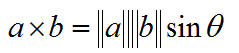使用行列式进行计算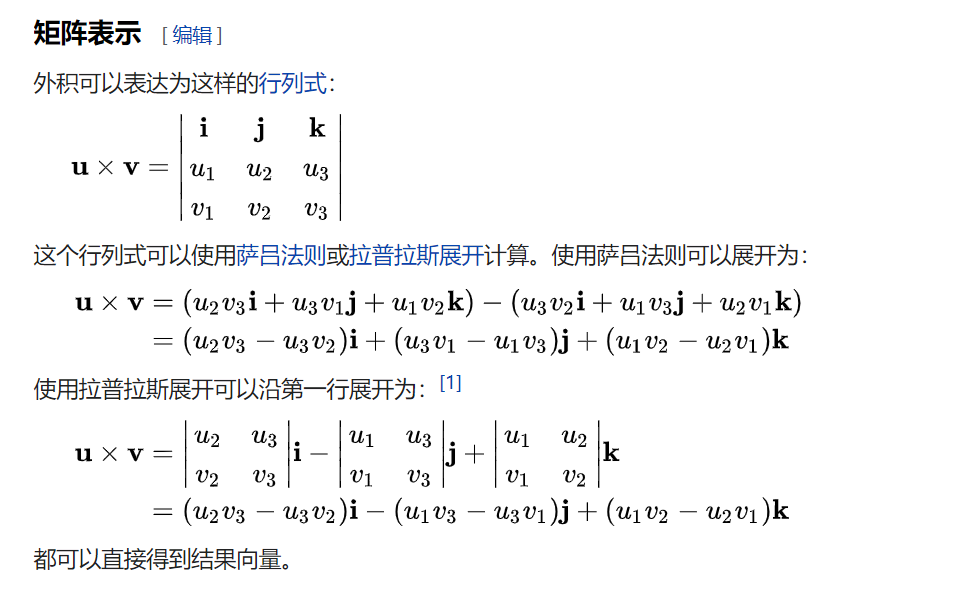4.向量叉乘方向的判断

向量的叉乘是通过右手定则来判断结果向量的方向的。伸出右手，四指弯曲符合向量叉乘的顺序，那么大拇指就是叉乘后结果向量的方向。如下图axb，右手四指弯曲方向从a到b，大拇指方向向上就是叉乘结果向量的方向。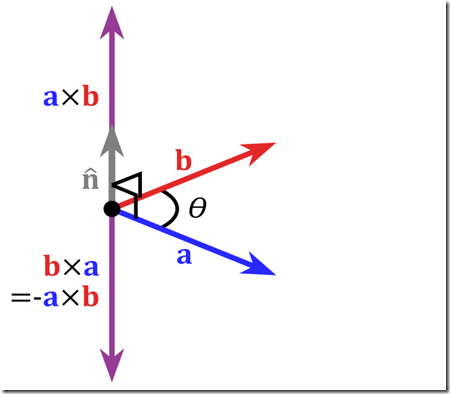原文：

https://www.cnblogs.com/metalsteel/p/6388062.html

展开全文• SVM支持向量机模型

万次阅读 2017-12-20 11:43:12
和感知机模型一样，SVM（支持向量机模型）也是旨在求出n维空间的最优超平面将正负类分开。这里的达到的最优是指在两类样本点距离超平面的最近距离达到最大，间隔最大使得它区别于感知机学习，SVM中还有核技巧，这样...

1.1SVM模型

和感知机模型一样，SVM（支持向量机模型）也是旨在求出n维空间的最优超平面将正负类分开。这里的达到的最优是指在两类样本点距离超平面的最近距离达到最大，间隔最大使得它区别于感知机学习，SVM中还有核技巧，这样SVM就是实际上的非线性分类器函数。

1.2线性可分支持向量机

跟前面定义的问题一样，假设给定一个特征空间上的训练数据集

T={(x1,y1),(x2,y2),(x3,y3),,(x1,y1)}

xiX=RnyiY={+1,1}i=1,2,3,,NNxiiyixi $x_i\in X=R^n，y_i\in Y=\{+1,-1\}，i=1,2,3,\cdots,N，表示N个样本实例，x_i为第i个特征向量（实例），y_i为x_i的类标记。$
目标是找到一个分离超平面，将正负类分别分到平面的两侧。分离超平面对应方程 wx+b=0 $w\cdot x+b=0$，当数据集是线性可分的时候，这样的超平面存在无数个，感知机利用误分类的点来求解，有无数个解。SVM利用间隔最大化求得最优超平面，解唯一。设分类决策函数为
f(x)=sign(wx+b)(1)

1.2.1函数间隔和几何间隔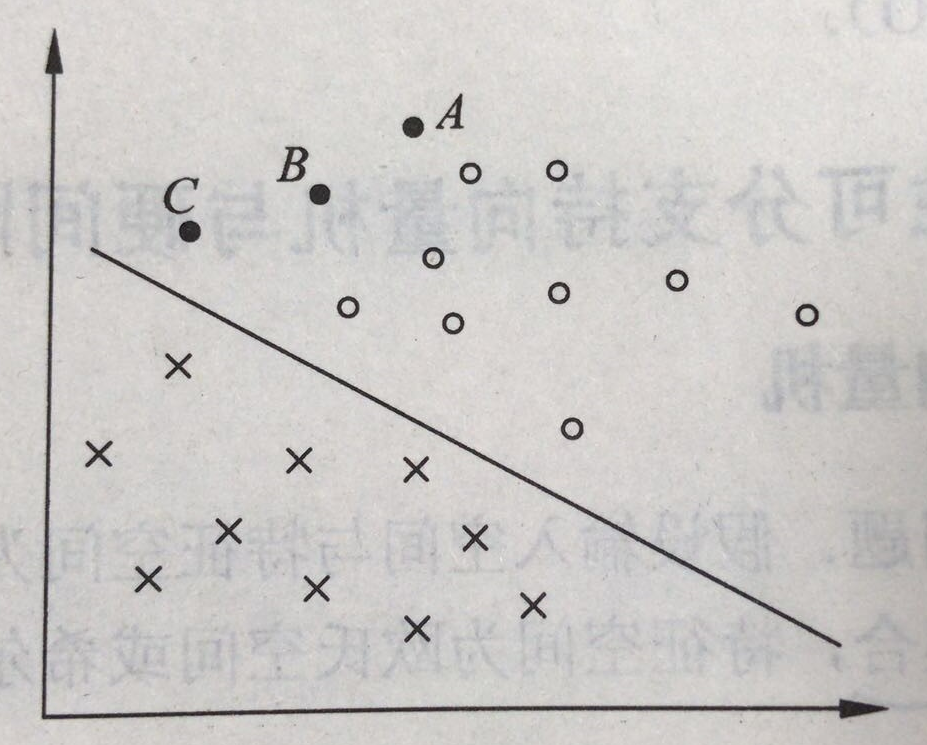上图中有A,B,C三个点，其中A点离超平面较远，将其决策为正类的确信度比较高，C点预测为正类的置信度就不是很高，相同的，B位于A,C之间，所以将其预测为正类的置信度也在点A和点C之间。一般来说，一个点距离超平面的远近决定了其分类结果的置信度，所以最优的平面即为离超平面最近的样本点到其的距离最大的时候。
给定样本点（ xi,yi $x_i,y_i$）,超平面（w,b）,超平面关于该样本点的函数间隔即为 yi(wxi+b) $y_i(w\cdot x_i+b)$，定义超平面关于整个数据集T的超平面的函数间隔为为超平面关于所有样本点的函数间隔最小值。函数间隔能有效表示分类预测的正确性及确信度
但是，对于一个超平面（w，x）来说，通过缩放变换（成倍的放大缩小w，b），超平面并没有改变，但是函数间隔却改变了。所以可以通过对超平面的法向量w加以约束，如规范化令||w||=1，这样间隔就是确定的，这时候的函数间隔即为几何间隔，即为点（ xi,yi $x_i,y_i$）到超平面（w,b）的距离

wxi+b||w||(2)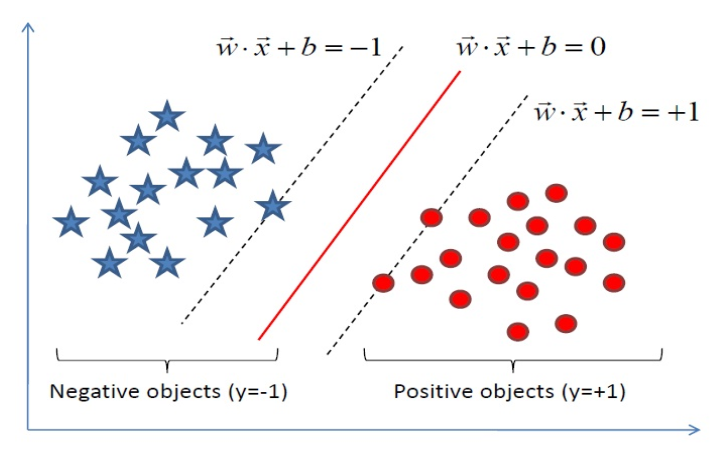因为y只取+1，-1，只影响符号，不影响数值。 但是几何间隔一般是指带符号的距离（李航老师书）上图中两条虚线之间的距离即为间隔
所以当||w||=1时，函数间隔和几何间隔相等，当w和b成倍放大缩小的时候，函数间隔也会按照比例改变，几何间隔是不变的。
总结一下：函数间隔即为即为 yi(wxi+b) $y_i(w\cdot x_i+b)$，但是在w,b成倍缩放情况下，超平面不变，函数间隔不稳定，加个约束，几何上的点到直线的距离 wxi+b||w|| $\frac{w\cdot x_i+b}{||w||}$加上符号以后： yi(wxi+b)||w|| $\frac{y_i(w\cdot x_i+b)}{||w||}$为几何间隔，为了便于求得最优解，我们通常通过缩放变换使得函数间隔为1。所以SVM求解的目标函数为函数间隔为1情况下的几何间隔。

1.2.2 SVM学习算法

支持向量机的目的在于求得最优的即几何间隔最大的超平面，在样本数据是线性可分的时候，这里的间隔最大化又叫硬间隔最大化（训练数据近似可分的话就叫软间隔）
支持向量机的学习算法可以表示为下面的约束最优化问题：

maxw,bΥ=wxi+b||w||

s.t. yi(wx+b)1,i=1,2,3,,N

前面有提到，可以通过缩放变换（w,b）改变函数间隔的大小，但是超平面不改变，这里我们可以使函数间隔为1，这样问题变为
maxw,bΥ=1||w||

s.t. yi(wx+b)1,i=1,2,3,,N

很多书的分子是2， 训练集样本点中距离超平面最近的样本称为支持向量，因为存在正负类的支持向量，所以double一下，这里对求得最后最优解并不影响。 值得指出的是，决定分离超平面的时候只有支持向量起作用，因为他们决定了函数间隔和几何间隔，其他点不起作用。
求解 maxw,b1||w|| $\mathop{max}_{w,b}\frac{1}{||w||}$,既是求
minw,b12||w||2(3)

s.t. yi(wx+b)1,i=1,2,3,,N

这就是支持向量机的目标函数，这是一个 凸二次规划问题，所以支持向量机的学习算法又叫 最大间隔法。那么该如何求得在约束条件下最优的超平面的参数(w,b)呢？

1.2.3 SVM对偶算法

SVM通过对其对偶问题的求解求得最优的超平面参数(w,b)，对于目标函数(12)，目标函数是二次的，约束条件是线性的，是一个标准的QP问题，但是可以通过拉格朗日对偶性求得对偶问题的最优解，一者，这样更高效，二者还可以自然引入核函数，推广到非线性的分类问题。
首先构建拉格朗日函数，对每一个约束条件引进拉格朗日乘子 αi0,i=1,2,3,,N $\alpha_i\ge0,i=1,2,3,\cdots,N$，定义拉格朗日函数：

L(w,b,α)=12||w||2+i=1Nαi(1yi(wxi+b))(4)

其中的 α=α1,α2,,αn $\alpha={\alpha_1,\alpha_2,\cdots,\alpha_n}$为拉格朗日乘子向量。我们令：
θ(w)=maxαi0L(w,b,α)

对于式子(4)来说，要是存在某个样本不满足条件 yi(wx+b)1 $y_i(w\cdot x+b)\geq1$，那么 θ= $\theta=\infty$,(只要令 αi= $\alpha_i=\infty$就可以)，若是所有条件都满足，则有 θ(w,b)=12||w2|| $\theta(w,b)=\frac{1}{2}||w^2||$，即令后面减掉的数为零即可获得最大值。保证减号后面的数字为0，当 1yi(wxi+b)=0 $1-y_i(w\cdot x_i+b)=0$，即为点为支持向量(最靠近超平面的点)时， /alphai $/alpha_i$可以为任意值，当 1yi(wxi+b)>0 $1-y_i(w\cdot x_i+b)\gt0$时，非支持向量时， /alphai=0 $/alpha_i=0$，这里可以解释为什么SVM只跟支持向量有关。这样我们的目标函数变成了：
minw,bθ(w,b)=minw,bmaxαi0L(w,b,α)=p

p $p^*$表示要求的最优解，和我们最初要求的问题是等价的。交换一下最大最小的位置：
maxαi0minw,bL(w,b,α)=d

交换之后的解 d $d_*$不等价于原问题，和 p $p^*$是对偶问题，并且有 dp $d_*\le p^*$，直观解释就是最大值中最小的总比最小值中的最大值要大，在满足某些条件的时候，两者相等，这里的条件即为 KKT条件

将公式（4）后面括号展开，就得到

L(w,b,α)=12||w||2i=1Nαiyi(wxi+b)+i=1Nαi(5)

对w,b分别求导,
Lw=wNi=1αixiyi

Lb=Ni=1αiyi

并令其等于0：
w=Ni=1αiyixi

Ni=1αiyi=0

带入公式5，得：
L(w,b,α)=i=1Nαi12i=1Nj=1NαiαjyiyjxTixj(6)

这样，所求目标函数变为：
maxαi(i=1Nαi12i=1Nj=1NαiαjyiyjxTixj)(7)

s.t.i=1Nαiji=0

αi0

对于上式，可以看出，求出 α $\alpha$便求出了超平面w,b：
w=i=1Nαiyixi

b=maxi:yi=1wxi+mini:y=+1wxi2

求解公式7中的 α $\alpha$，可以用著名的 SMO算法

2.核函数

上文提到，在求解出 w=Ni=1αiyixi $w=\sum_{i=1}^{N}\alpha_iy_ix_i$后，对于一个新的点x，带入超平面方程，得

y=(i=1Nαiyixi)x+b=i=1Nαiji<xix>+b(8)

这样每预测一个新的点x时，只需要计算它与训练样本中的点的内积，这是引入核函数的重要前提。这里与之求内积的就是支持向量，非支持向量的 α $\alpha$系数为0。

2.1.非线性分类问题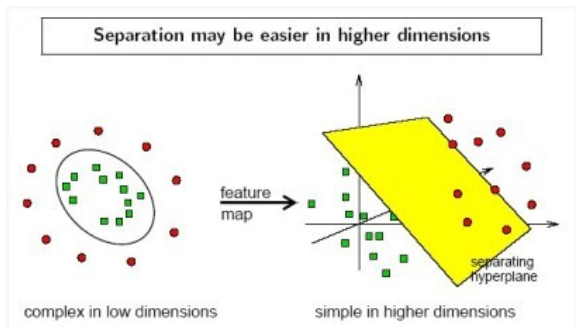上图所示，在左边低维度上，只能靠一个非线性平面（椭圆）将正负类分开，映射到高维(右图)，可以看到在高维度下可以找到这样一个超平面。这就是非线性可分的。也就意味着，在我们遇到核函数之前，面对这类问题，需要完成两步：1.首先使用一个非线性映射将数据变换到一个特征空间F，2. 然后在特征空间使用线性学习器分类。分类决策函数如下：

f(x)=i=1NwiΦ(xi)+b

其中， Φx $\Phi（x）$就是将样本映射到高维空间的映射函数。

2.2.核函数

在上文我提到过对偶形式，而这个对偶形式就是线性学习器的一个重要性质，这意味着假设超平面可以表达为训练点的线性组合，因此决策规则可以用测试点和训练点的内积来表示：

f(x)=i=1Nαiyi<Φ(xi),Φ(x)>+b

其中 <Φ(xi),Φ(x)> <script type="math/tex" id="MathJax-Element-337"><\Phi (x_i),\Phi (x)></script>表示两个向量的内积，即上文中的点乘符号。如果存在一种计算方式，能够在当前低维的特种空间直接计算 <Φ(xi),Φ(x)> <script type="math/tex" id="MathJax-Element-338"><\Phi (x_i),\Phi (x)></script>，那么上述的计算非线性可分问题的两个步骤就能合成一步建立一个非线性的分类器。这个就是核函数：
K<x1,x2>=<Φ(x1),Φ(x2)>

李航老师的《机器学习》中：对偶形式的基本思想是，将w,b表示为实例x和标记y的线性组合，求解该线性组合的系数而求得w,b。有了核函数，就不用关心映射之后的维度有多少（有些甚至是无限维，例如高斯核）。

2.2.线性不可分和软间隔最大化

并不是所有的样本都是线性可分或者非线性可分的，面对不可分的情况，即公式3中的约束条件不成立。可以引进一个松弛变量 ξi0 $\xi_i\ge0$,使得函数间隔加上松弛变量大于等于1，这样公式3约束条件就变为：

yi(wxi+b)1ξi

每个松弛变量 ξi $\xi_i$给一个代价，目标函数变为：
12||w||2+Ci=1Nξi

s.t.yi(wxi+b)1ξi,i=1,2,,N

ξ0,i=1,2,,N

C为惩罚系数。之后的推导和对偶问题同上。

展开全文svm
• 词向量经典模型：从word2vec、glove、ELMo到BERT

万次阅读 多人点赞 2018-12-05 23:39:27
向量技术将自然语言中的词转化为稠密的向量，相似的词会有相似的向量表示，这样的转化方便挖掘文字中词语和句子之间的特征。生成词向量的方法从一开始基于统计学的方法（共现矩阵、SVD分解）到基于不同结构的神经...

前言

词向量技术将自然语言中的词转化为稠密的向量，相似的词会有相似的向量表示，这样的转化方便挖掘文字中词语和句子之间的特征。生成词向量的方法从一开始基于统计学的方法（共现矩阵、SVD分解）到基于不同结构的神经网络的语言模型方法。这里总结一下比较经典的语言模型方法：word2vec、glove、ELMo、BERT。

word2vec

word2vec来源于2013年的论文《Efficient Estimation of Word Representation in Vector Space》，它的核心思想是通过词的上下文得到词的向量化表示，有两种方法：CBOW（通过附近词预测中心词）、Skip-gram（通过中心词预测附近的词）：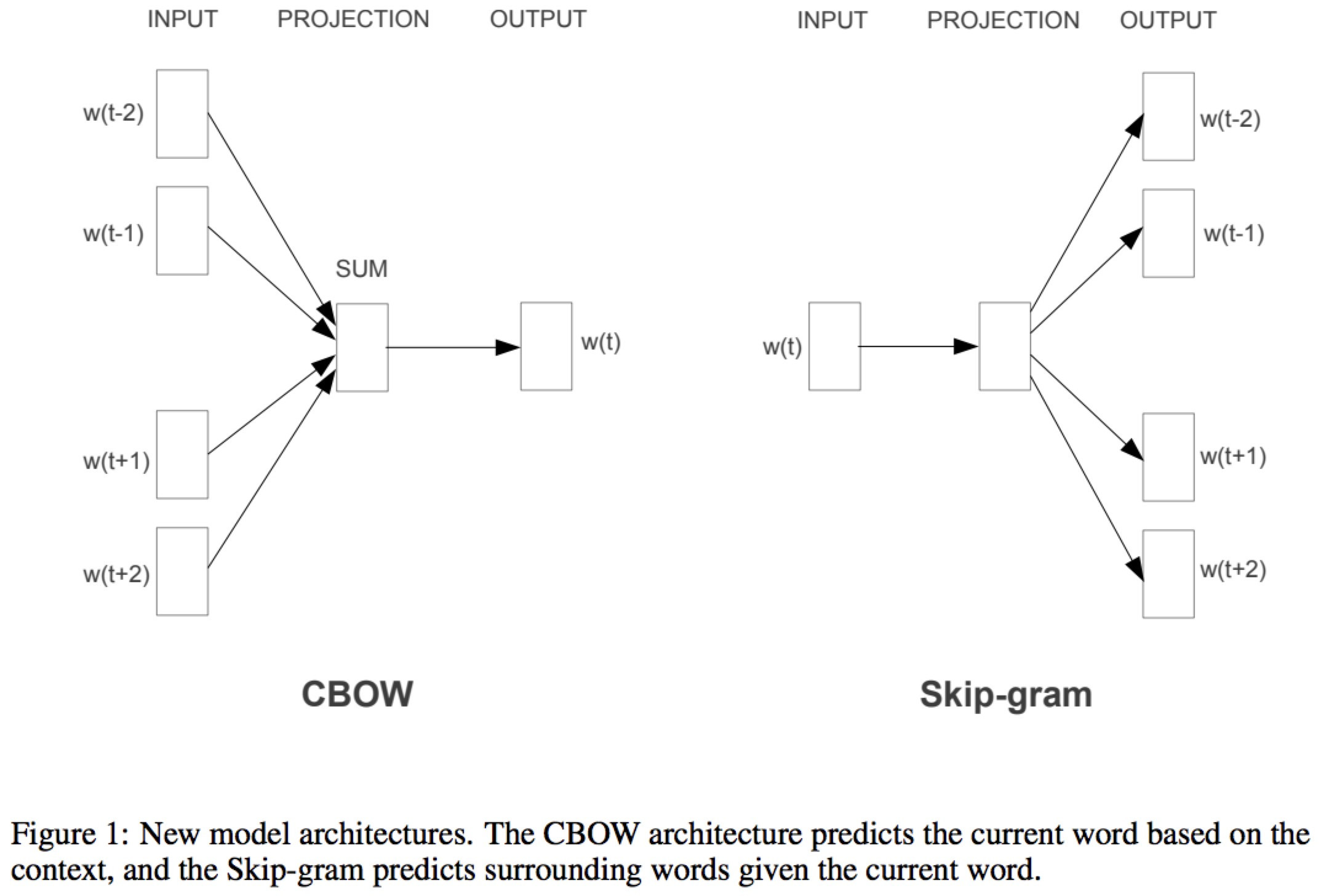CBOW :
通过目标词的上下文的词预测目标词，图中就是取大小为2的窗口，通过目标词前后两个词预测目标词。具体的做法是，设定词向量的维度d，对所有的词随机初始化为一个d维的向量，然后要对上下文所有的词向量编码得到一个隐藏层的向量，通过这个隐藏层的向量预测目标词，CBOW中的做法是简单的相加，然后做一个softmax的分类，例如词汇表中一个有V个不同的词，就是隐藏层d维的向量乘以一个W矩阵（ R d × V \mathbb{R}^{d \times V} ）转化为一个V维的向量，然后做一个softmax的分类。由于V词汇的数量一般是很大的，每次训练都要更新整个W矩阵计算量会很大，同时这是一个样本不均衡的问题，不同的词的出现次数会有很大的差异，所以论文中采用了两种不同的优化方法多层Softmax和负采样。
Skip-gram :
跟CBOW的原理相似，它的输入是目标词，先是将目标词映射为一个隐藏层向量，根据这个向量预测目标词上下文两个词，因为词汇表大和样本不均衡，同样也会采用多层softmax或负采样优化。
多层softmax :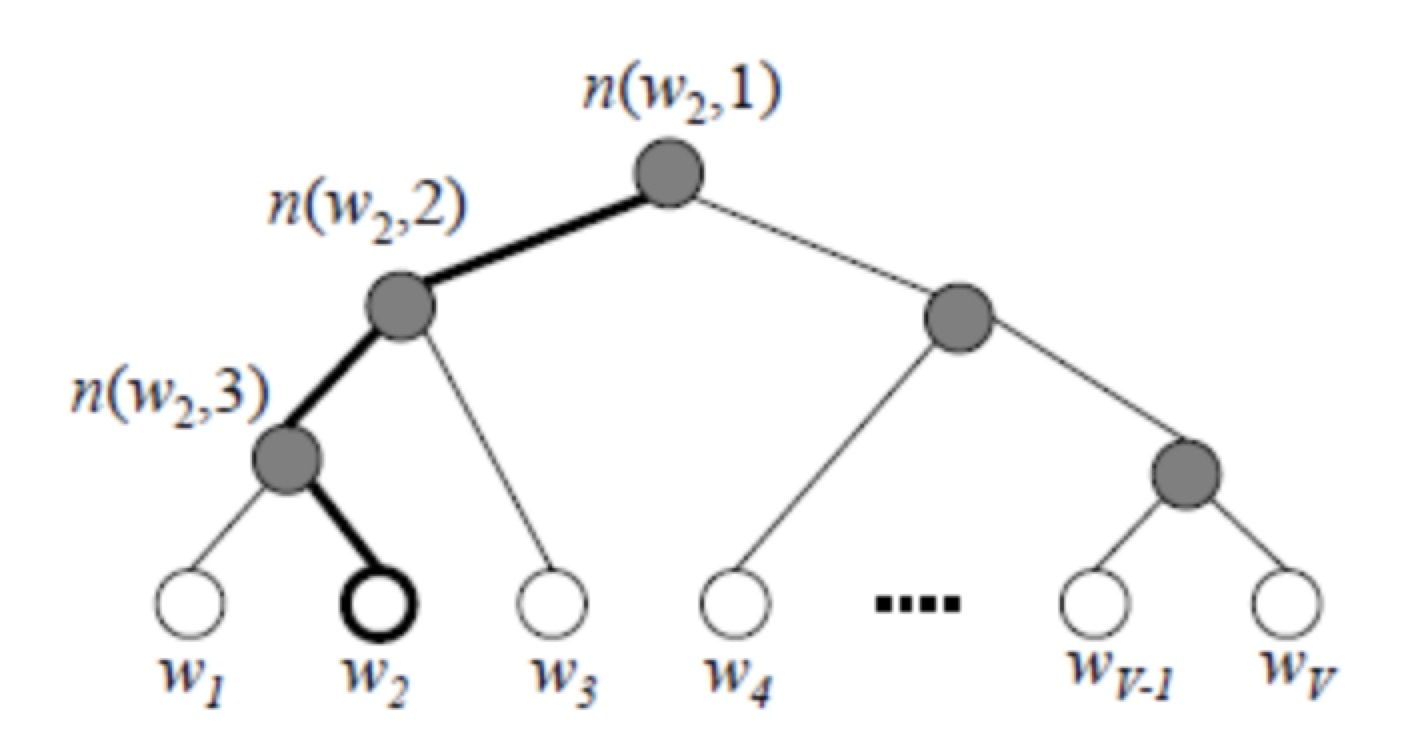由于单词出现的频率是不一样的，所以用哈夫曼编码构建一个二叉树，出现频率高的词放在前面可以减少计算量，用哈夫曼编码记录路径，例如图中单词2（ w 2 w_2 ，规定左边走是1，右边走是0）的哈夫曼编码就是1110，在路径上每到一个节点就是一个sigmoid的二分类，所以叫多层softmax。具体来说每个节点处会有一个d维的向量参数 θ \theta ，每个单词也是d维的向量 x w x_w ，一个sigmoid的方程决定向左或者右走的概率，往左走的概率是：
P ( + ) = σ ( x w T θ ) = 1 1 + e − x w T θ P(+) = \sigma(x_w^T\theta) = \frac{1}{1+e^{-x_w^T\theta}}
将路径上的概率连乘，极大化这个概率值，就是模型的训练目标，每次用一个样本更新梯度，使用对数似然函数：
L = ∑ j = 2 l w ( 1 − d j w ) l o g [ s i g m a ( x w T θ j − 1 w ) ] + d j w l o g [ 1 − s i g m a ( x w T θ j − 1 w ) ] L = \sum^{l_w}_{j=2}(1 - d_j^w)log[sigma(x_w^T\theta^w_{j-1})] + d_j^wlog[1 - sigma(x_w^T\theta^w_{j-1})]
其中 l w l_w 代表目标词在哈夫曼树中的深度， d j w d_j^w 代表在j层中目标词根据哈夫曼编码的路径应该向左还是向右走，左是1右是0，可以看到整个目标函数跟逻辑回归是基本相似的，梯度的计算也跟逻辑回归基本一样。
对于CBOW，多层softmax是将窗口内的2c个词求平均得到 x w x_w ，放入哈夫曼树的网络中，每一层得到的梯度更新哈夫曼树中的参数，同时梯度同步更新到2c个单词的向量中。
对于Skip-gram，多层softmax是用目标词的词向量为 x w x_w ，然后遍历窗口内的2c个词，一个进行2c次的训练，每次将 x w x_w 放进哈夫曼树中，更新 x w x_w 和树中的参数。
负采样 :
负采样也是把模型变为二元的逻辑回归，每个词对应一个词向量 x w x_w 和词的网络参数 θ w \theta_w ，每个词附近有2c个词，通过负采样，随机采样neg个反例，让正例词对目标词的词向量和窗口内词的网络参数的乘积尽可能的大，反例的尽可能小，是模型的训练目标。例如在skip-gram中目标词A的词向量是 x A x_A ，它2c的窗口内附近一个词的网络参数是 θ B \theta_B ，让这两个的乘积尽可能的大， σ ( x A T θ B ) \sigma(x_A^T \theta_B) 尽可能大，反例，随机采样出来的词乘积尽可能小。同理CBOW中是将2c个词相加求平均，然后与目标词的网络参数相乘，也是让窗口内真实存在的词乘积尽可能大，随机采样出的词尽可能小。
负采样的方式是根据词出现的频率进行采样，出现频率越高，越可能被采样到，原文中是根据出现频率的3/4次方然后做一个归一化作为概率进行采样。

另外这篇论文的作者在2016年开源了一个文本分类的工具，FastText，与word2vec的原理非常类似，只不过在做文本分类时是一个有监督的分类预测文本的label。另外加入n-gram的特征，对于英文，每个单词前后加入标识，例如#good#，如果是3-gram的特征就是加入#go,goo,ood,od#，可以解决一些oov的问题（不在训练集中出现的生词）和引入一些词根的特征。对于中文“你爱我”和“我爱你”有完全不同的语义，用CNN或者highway网络处理的char embedding可以引入这部分的特征。

glove

word2vec只考虑到了词的局部信息，没有考虑到词与局部窗口外词的联系，glove利用共现矩阵，同时考虑了局部信息和整体的信息。来自论文《Glove: Global vectors for word representation》。
不知道为什么大家都用这个例子：i love you but you love him i am sad。。。可能程序员的现实就是这么残酷。。。
用这个例子介绍窗口，其实跟word2vec是一样的。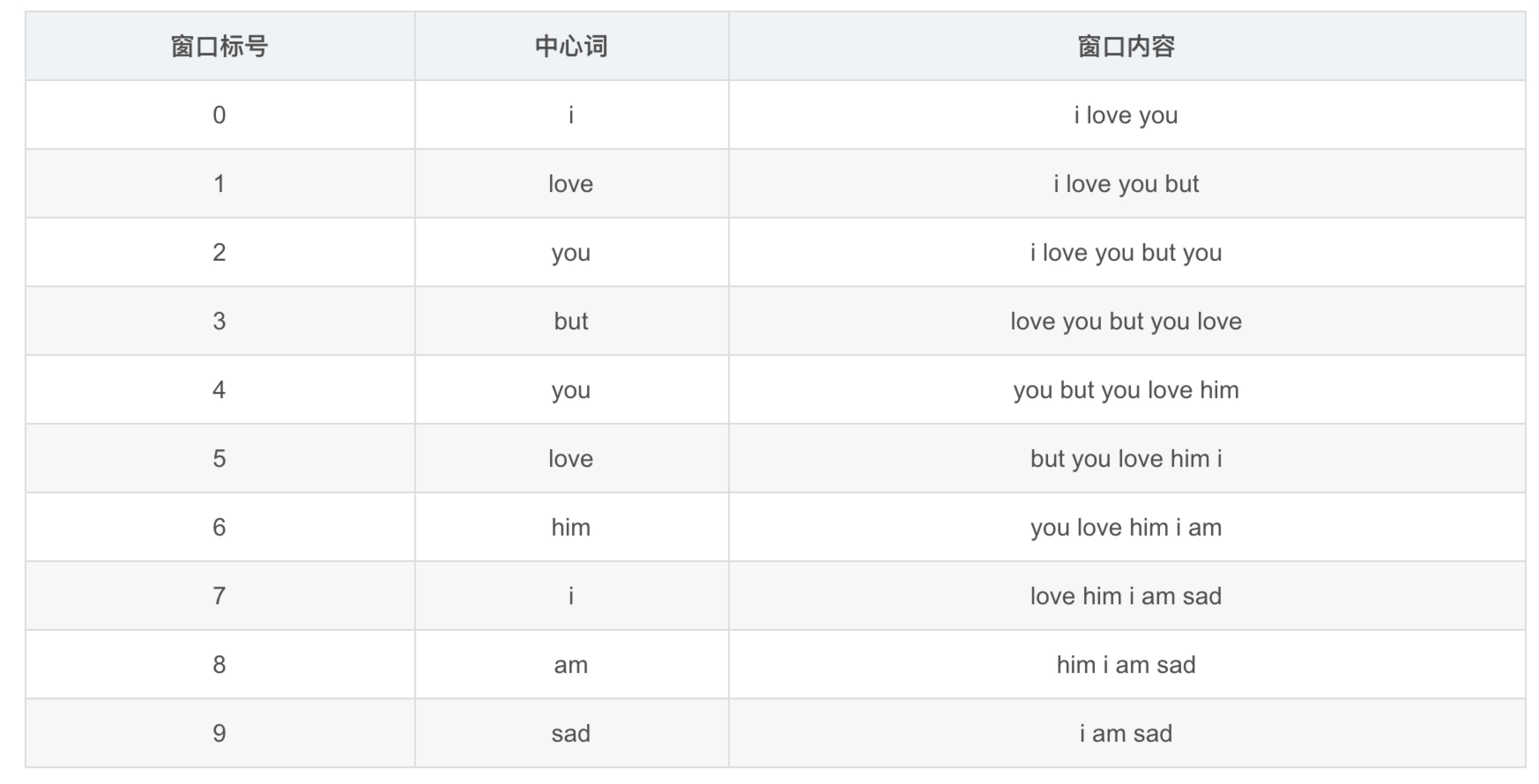作者定义了几个符号：
1、 X i , j X_{i,j}
它是通过遍历所有的窗口内容统计得到的， X i , j X_{i,j} 表示以i为中心词，j出现在窗口内容中的次数。如上图所示，例如love的窗口内容是but、you、him、i，那么 X l o v e , b u t ， X l o v e , y o u ， X l o v e , h i m ， X l o v e , i X_{love, but}，X_{love, you}，X_{love, him}，X_{love, i} 都加1。
2、 X i X_i
X i = ∑ j = 1 N X i , j X_i = \sum^N_{j=1} X_{i, j}
也就是统计词i一共是多少个其他词的中心词。
3、 P i , k P_{i,k}
P i , k = X i , k X i P_{i,k} = \frac{X_{i,k}}{X_i}
也就是词k在词i附近占词i所有的附近词的比例。
4、 R a t i o i , j , k Ratio_{i,j,k} :
R a t i o i , j , k = P i , k P j , k Ratio_{i,j,k} = \frac{P_{i,k}}{P_{j,k}}
也就是词k出现在词i和出现在词j附近的比例的比例。。。
然后作者发现一个规律：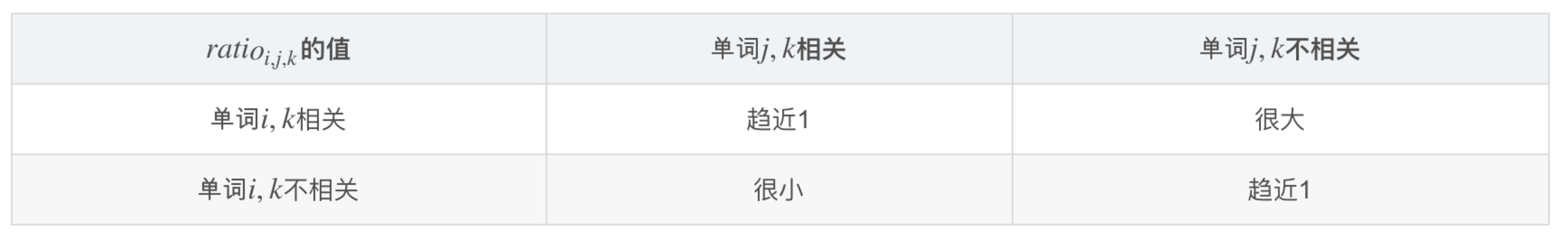然后作者就设计目标函数去拟合这个规律，这个ratio跟i,j,k有关，所以将词i,j,k的向量放进函数中，代价函数的模版应该是：
J = ∑ i , j , k N ( P i , k P j , k − g ( v i , v j , v k ) ） J = \sum^N_{i,j,k} (\frac{P_{i,k}}{P_j,k} - g(v_i, v_j, v_k)）
重点就在于如何设计g(*)这个函数，首先，它是比较 v i , v j v_i,v_j v k v_k 附近的比例的差异，因为比较差异所以应该有一项减法 ( v i − v j ) (v_i - v_j) ，然后是在 v k v_k 附近时候它们的差异，所以应该跟 v k v_k 有关，同时ratio是一个标量，要将差异向量转化为一个标量所以变为 ( v i − v j ) T v k (v_i - v_j)^T v_k ，然后要做一个变换使它符合ratio的特点，当 v i , v j v_i,v_j v k v_k 附近出现比例的比例是相似时候，ratio应该约等于1，也就是 ( v i − v j ) (v_i - v_j) 约等于0的时候， g ( v i , v j , v k ) g(v_i, v_j, v_k) 应该约等于1，同时应该满足 g ( v i , v j , v k ) g(v_i, v_j, v_k) v i v_i (对应 P i , k P_{i,k} )的关系是单调递增，跟 v j v_j (对应 P i , j P_{i,j} )的关系是单调递减的，这时候exp()函数符合这个规律，所以最后的损失函数变为：
P i , k P j , k = e x p ( ( v i − v j ) T v k ) \frac{P_{i,k}}{P_j,k} = exp((v_i - v_j)^Tv_k)
经过一些数学的变换变为：
P i , k P j , k = e x p ( v i T v k ) e x p ( v j T v k ) \frac{P_{i,k}}{P_j,k} = \frac{exp(v_i^Tv_k)}{exp(v_j^Tv_k)}
这里如果整个损失函数更新时间复杂度是 O ( n 3 ) O(n^3) ，如果等式两边对应分子等于分子，分母等于分母可以让复杂度将为 O ( n 2 ) O(n^2) 。两边取对数得到：
P i , j = e x p ( v i T v j ) P_{i,j} = exp(v_i^Tv_j)
但是存在一个问题， P i , j P_{i,j} P j , i P_{j,i} 是不等的，但是 e x p ( v i T v j ) exp(v_i^Tv_j) e x p ( v j T v i ) exp(v_j^Tv_i) 却是相等的，留意到 X i , j X_{i,j} X j , i X_{j,i} 是相等的，可以把 P i , j P_{i,j} 展开，等式写为：
l o g ( X i , j ) − l o g ( X i ) = v i T v j log(X_{i,j}) - log(X_i) = v_i^Tv_j
加入偏置项，并把 l o g ( X i ) log(X_i) 并入，最后代价方程变为：
J = ∑ i , j N ( v i T v j + b i + b j − l o g ( X i , j ) ) 2 J = \sum^N_{i,j} (v_i^Tv_j+ b_i + b_j - log(X_{i,j}))^2
最小化代价方程得到词的向量表示。

ELMo

ELMo来自于论文《Deep contextualized word representations》，它的官网有开源的工具：https://allennlp.org/elmo

word2vec和glove存在一个问题，词在不同的语境下其实有不同的含义，而这两个模型词在不同语境下的向量表示是相同的，Elmo就是针对这一点进行了优化，作者认为ELMo有两个优势：
1、能够学习到单词用法的复杂特性
2、学习到这些复杂用法在不同上下文的变化
针对点1，作者是通过多层的stack LSTM去学习词的复杂用法，论文中的实验验证了作者的想法，不同层的output可以获得不同层次的词法特征。文中5.5章节有不同任务的情况对比，对于词义消歧有需求的任务，第2层会有较大的权重，对于对词性、句法有需求的任务对第1层会有比较大的权重。
针对点2，作者通过pre-train+fine tuning的方式实现，先在大语料库上进行pre-train，再在下游任务的语料库上进行fine tuning。

具体实现方式
ELMo来自于Embeddings from Language Models的简写，这里的language model作者采用了LSTM。一开始的训练目标可以表示为如下的形式：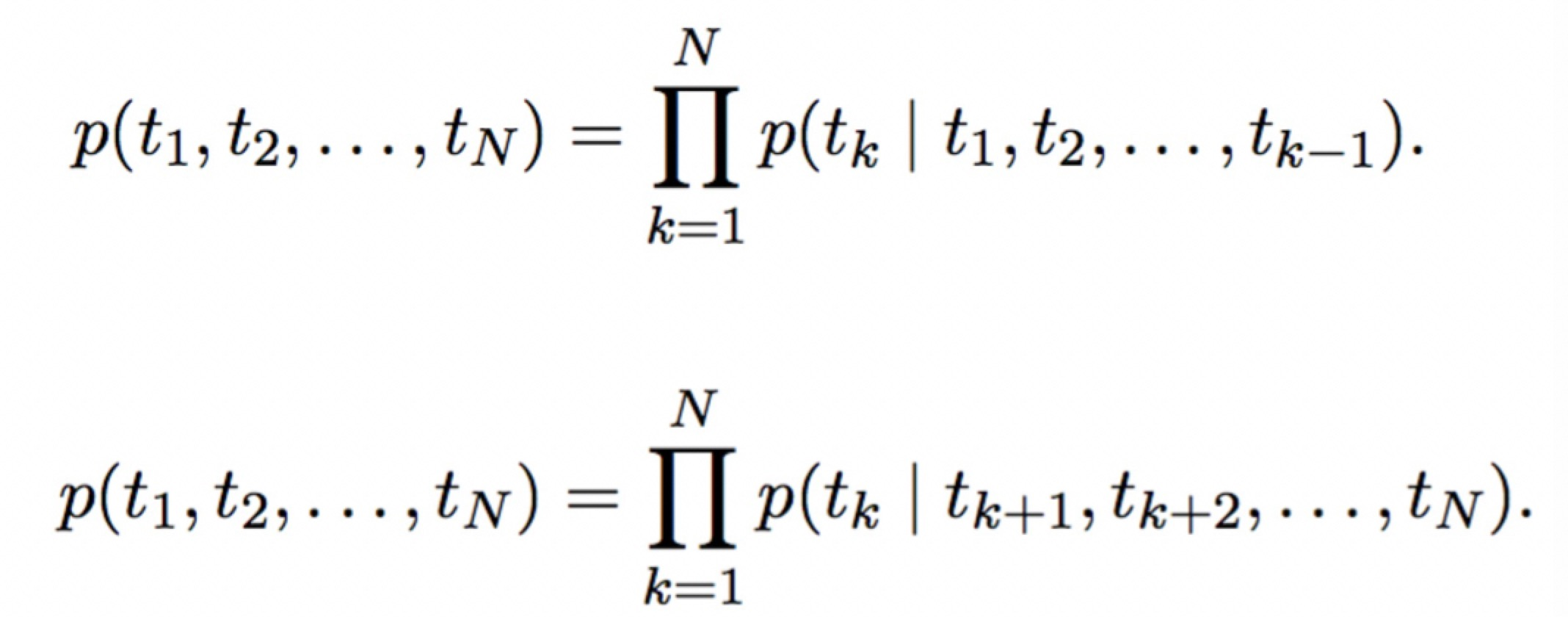具体来说是通过双方向预测单词，前向过程中，用1～k-1的词去预测第k个词，后向过程中，用k+1～N的词去预测第k个词。具体的编码方式作者采用了LSTM，可以表示为：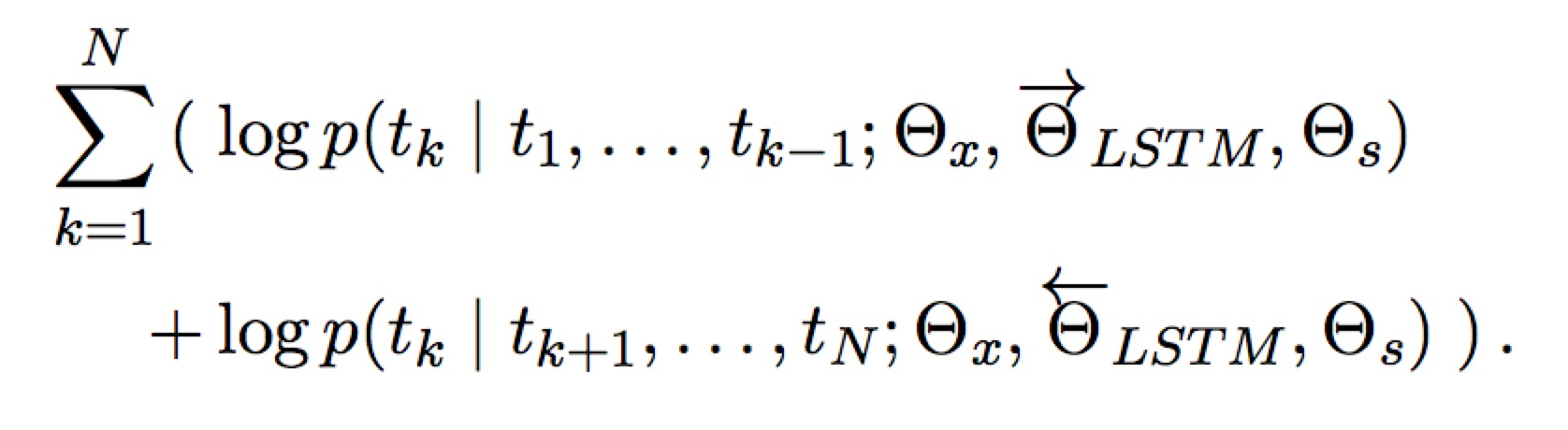通过LSTM编码Tokens，用第k-1个Token的隐藏层输出预测第k个Token，预测的方法是用一个softmax做一个分类，其中 Θ x \Theta_x 是token的向量表示， Θ s \Theta_s 是softmax的参数。这种双向LSTM堆叠L层，stack-RNN的方式也就是每一层隐藏层的输出作为下一层的输入。
那么对于每个Token会有2L+1个向量表示，双向所以每层2个，+1是token输出层的向量表示，作者将2L+1个向量组合起来得到ELMo每个token的向量表示：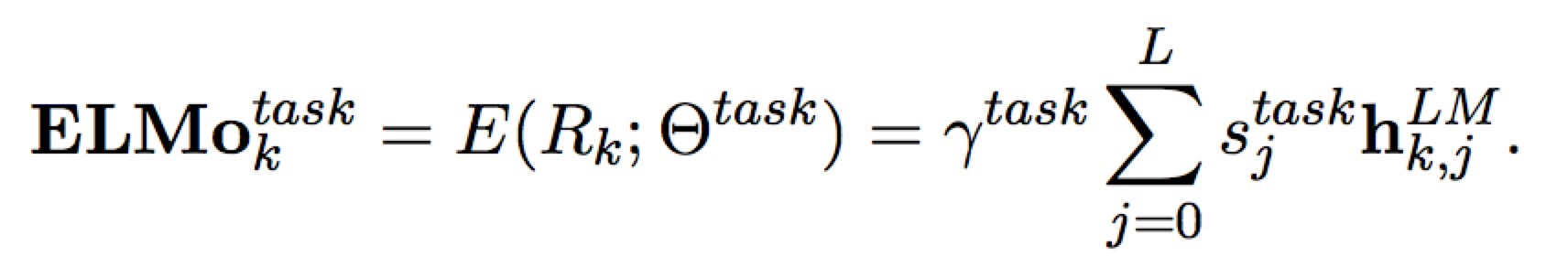其中 h k , j L M h_{k,j}^{LM} 是对于token k的第j层的隐藏层输出，当j=0的时候表示输入层token的向量表示，这个向量表示可以通过CNN或Highway等网络引入char-level的特征。 s j t a s k s_j^{task} 是一个softmax的归一化， γ t a s k \gamma^{task} 是一个放缩的参数，可以让目标模型对ELMo的向量进行放缩。
经过两层双向LSTM提取特征，又针对下游任务定制词向量，效果比word2vec和glove要好也在情理之中。

BERT

这里重点介绍一下BERT，它来自于googole发表的论文《BERT: Pre-training of Deep Bidirectional Transformers for Language Understanding》，这个模型彻底的改变了NLP的游戏规则。

改变游戏规则：

NLP一共有4大类的任务：

• 序列标注：分词／词性标注／命名实体识别…
• 分类任务：文本分类／情感分析…
• 句子关系判断：自然语言推理／深度文本匹配／问答系统…
• 生成式任务：机器翻译／文本摘要生成…

BERT为这4大类任务的前3个都设计了简单至极的下游接口，省去了各种花哨的Attention、stack等复杂的网络结构，且实验效果全面取得了大幅度的提升。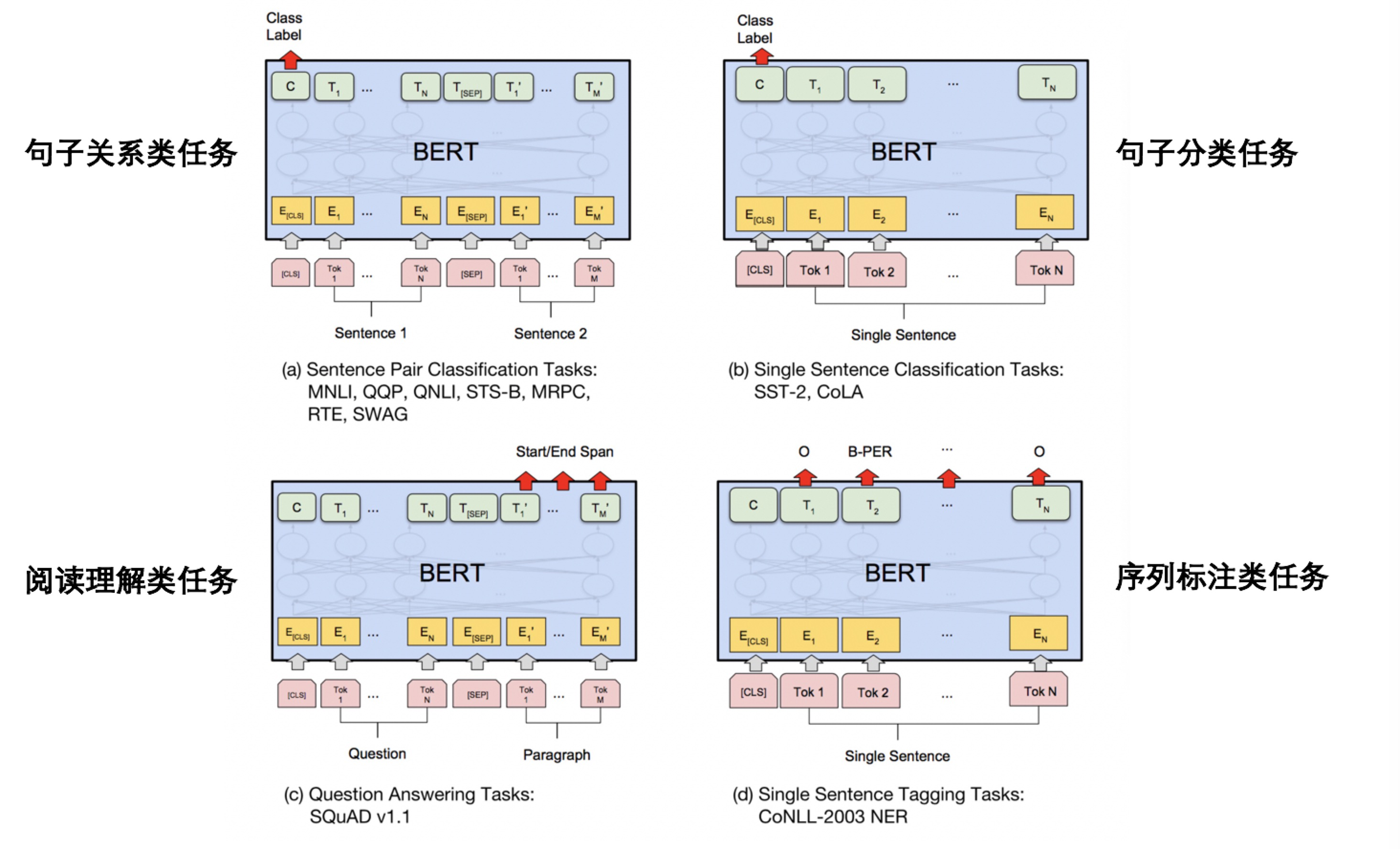上图是BERT论文中为下游任务设计的接口，可以先将BERT看作是一个黑盒，它跟ELMo的工作方式是类似的，都是现在大规模的语料库中pre-train，然后将下游任务输入，进行比较轻量级的fine-tuning。下游任务只要做一些轻微的改造就可以放入BERT的模型中fine-tuning，可以看到对于句子关系类的任务，只要加上句子起始和结束的符号，句子之间加入分割符号，然后经过BERT模型它最后的一个位置的输出连接上一个softmax的分类器就可以了。 对于序列标注的模型，也是只要加入起始和结束的符号，对于最后BERT每个位置的输出都加入一个线性的分类器就可以了。

BERT的诞生过程：

所以在ELMo之后，一个新模型GPT（来自论文《Improving Language Understanding by Generative Pre-Training》）推出了，它用Transformer来编码。但是它的推理方式跟ELMo相似，用前面的词去预测下一个词，所以它是单方向，损失掉了下文的信息。
然后BERT诞生了，它采用了Transformer进行编码，预测词的时候双向综合的考虑上下文特征。这里作者做了一个改进，原来没办法综合利用双向的特征是因为目标是用前面的词逐个的预测下一个词，如果你能看到另一个方向，那就等于偷看了答案。BERT的作者说受到了完形填空的启发，遮盖住文章中15%的词，用剩下85%的词预测这15%的词，那就可以放心的利用双向上下文的特征了。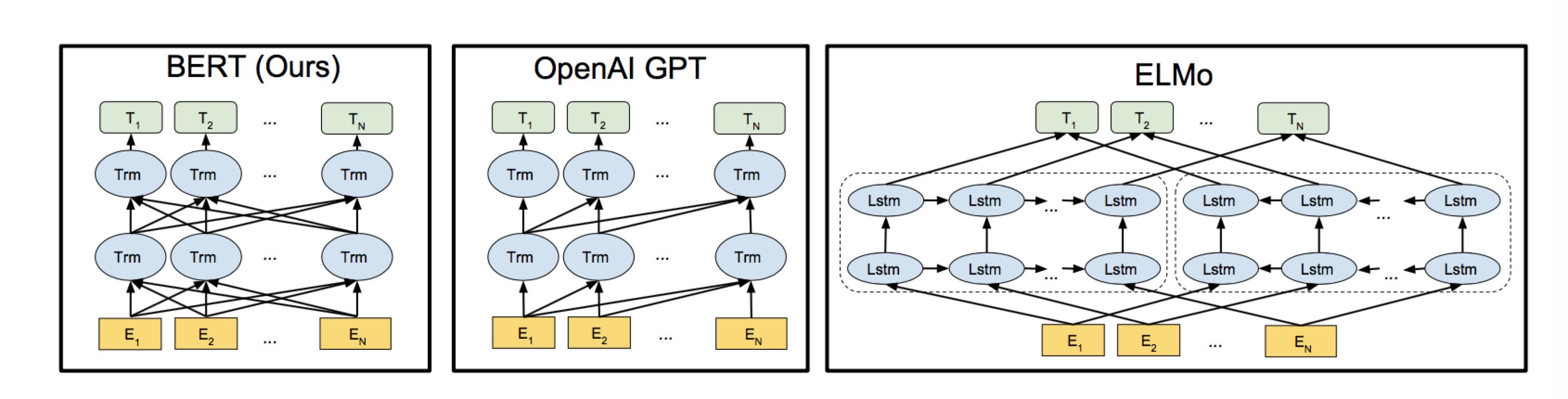Transformer：

Transformer是论文《Attention is all you need》中的模型，它把attention机制从配角搬上了主角的位置，没有采用CNN或者RNN的结构，完全使用attention进行编码。Transformer应该会取代CNN和RNN成为NLP主流的编码方式，CNN提取的是局部特征，但是对于文本数据，忽略了长距离的依赖，CNN在文本中的编码能力弱于RNN，而RNN是序列模型，并行能力差，计算缓慢，而且只能考虑一个方向上的信息。Transformer可以综合的考虑两个方向的信息，而且有非常好的并行性质，简单介绍一下Transformer的结构。

https://jalammar.github.io/illustrated-transformer/ 这一篇博客上有非常详细的介绍，详细了解Transformer可以看一下这一个博客。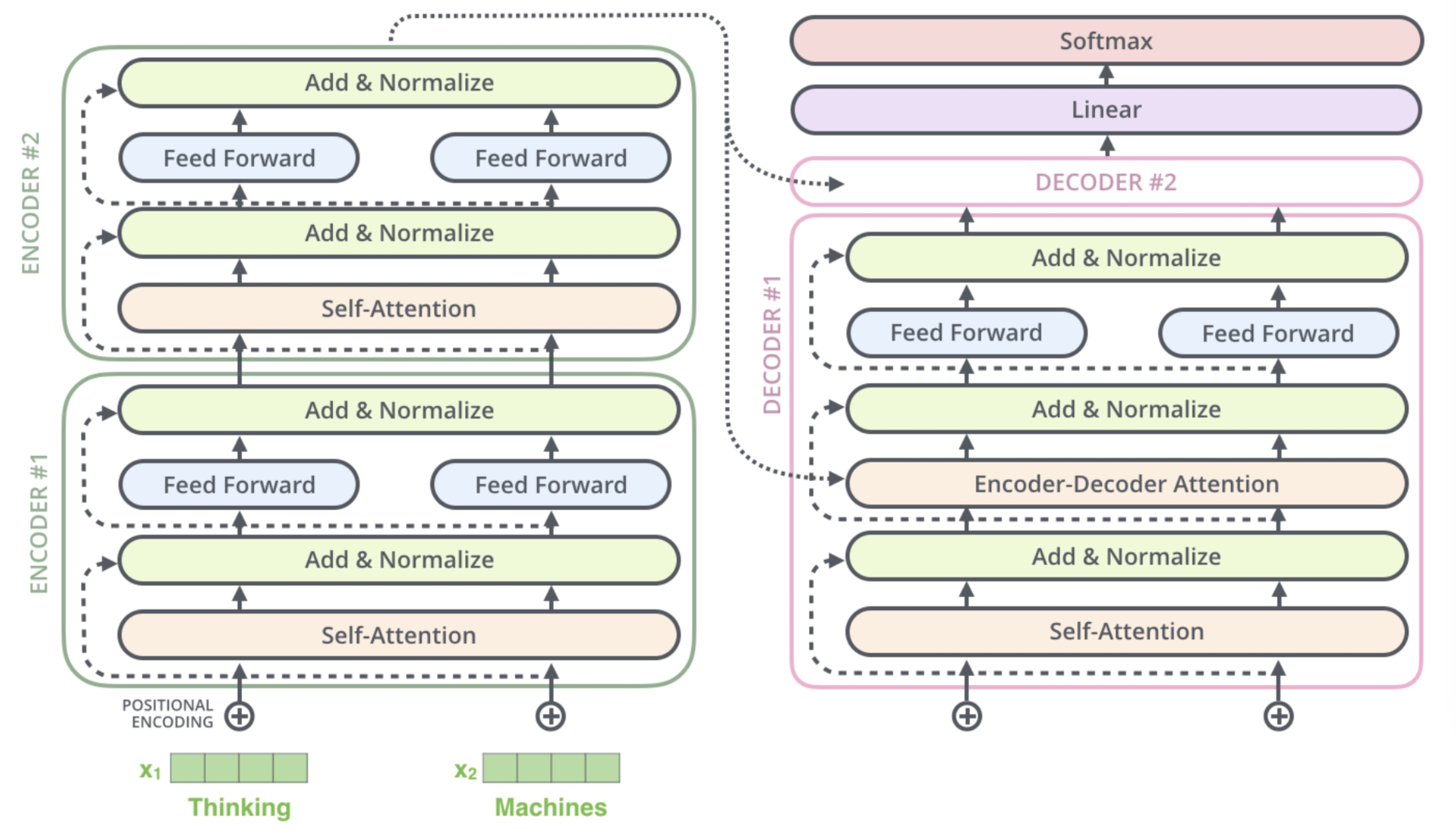整个Transformer的结构如上图所示，它分为两个部分，一边是encoder，另一边是decoder，一个encoder或decoder看作一个block，encoder和decoder又有多个block串行相连，图中是2个，原文中使用了6个。
先看encoder，单独看一个block，输入一行句子，先经过一个Self-Attention，Self-Attention首先是对每个Token的embedding通过3个不同的矩阵映射成3个向量，Query(Q)、Key(K)和Value(V)。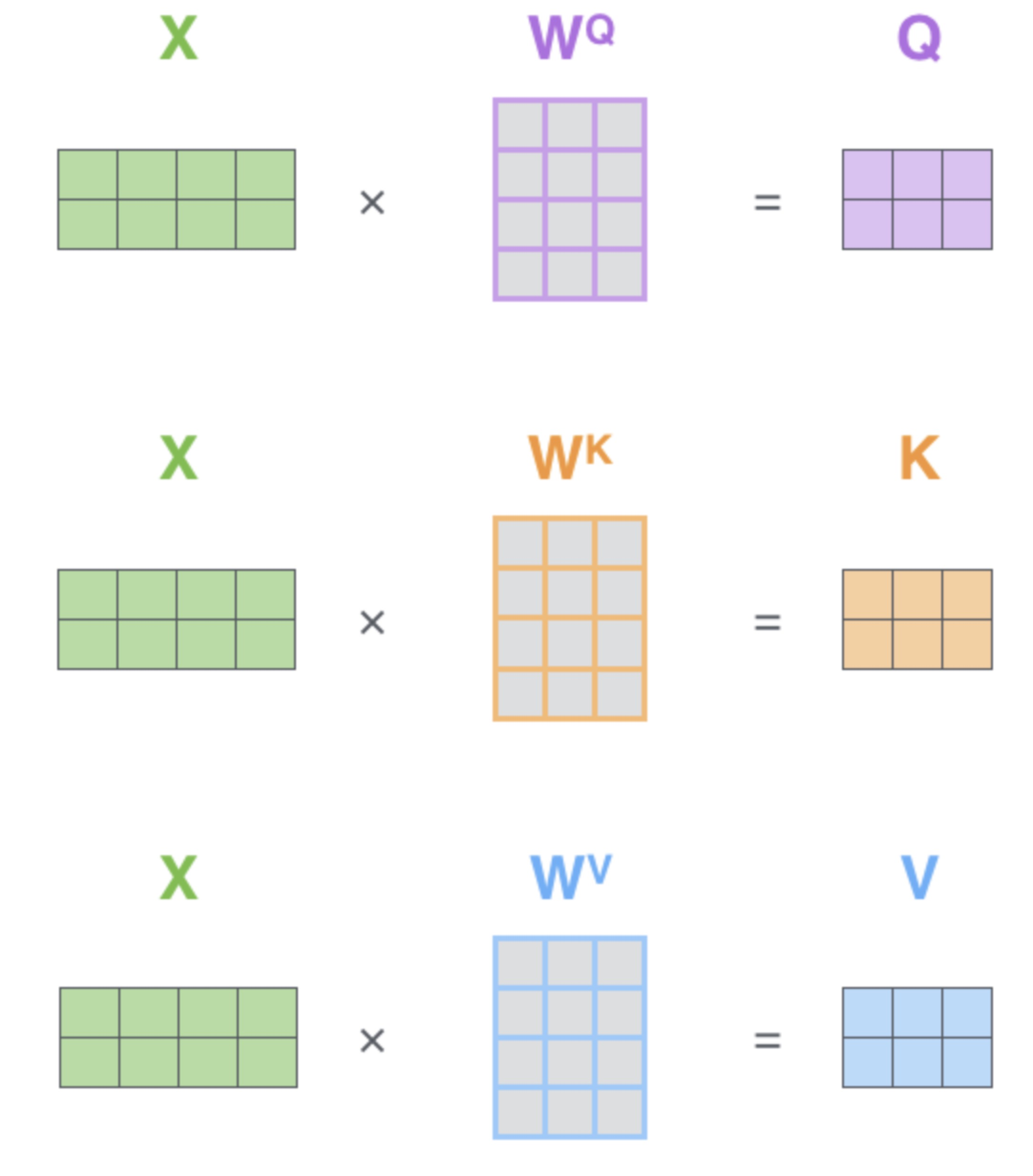上图是假设句子的长度只有2个tokens，由于Q、K、V的三个W转换矩阵在不同的位置是共享参数的，所以可以使用矩阵运算。
然后Q和K向量是用于计算Attention的权重参数的，计算出的权重参数乘到V向量上，再加权求和就得到每个token的向量。计算的过程是这样的：例如要计算位置1的向量，首先Q1和K1到Kn点乘，得到n个标量，这个标量除以 d k \sqrt{d_k} d k d_k 是K的向量的维度，然后做一个softmax的归一化，然后归一化后的权重对应乘到V1到Vn上，求和得到位置1的Token的向量。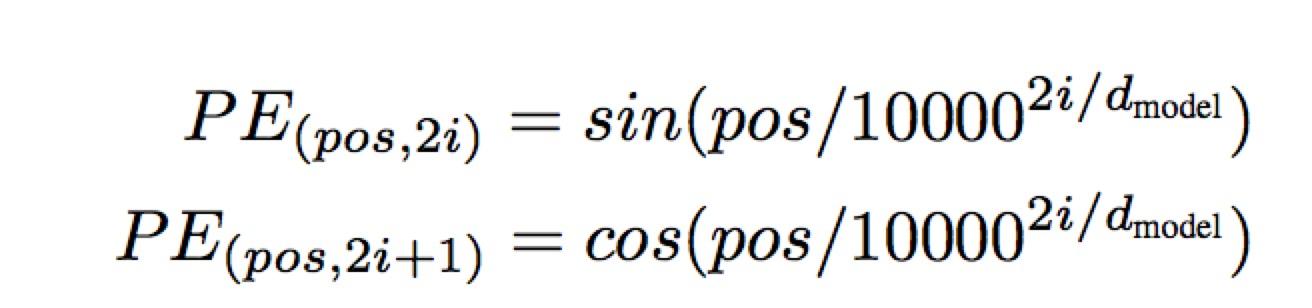每个pos的位置用一个d维的向量表示，这个向量的偶数位置用sin，奇数位置用cos计算，得到-1到1之间的值，之所以用三角函数是利用了三角函数和差变换可以线性变换的特性，因为BERT中没有采用这种计算方式，所以不作详细的介绍。

BERT的实现方式：

• 10%的词保留原来真实的词
• 10%的词用随机的一个词替代

编码方式：
BERT的Transformer结构与Attention is all you need中有一个不同是对于位置信息的编码，论文中的Transformer是采用cos和sin函数计算，而BERT用了更为简单粗暴的方法，每一个位置赋予一个向量，例如句子的截断长度固定为50，那么0-49这50个位置各赋予一个向量，将这个向量加到self-attention的embedding上。

获取句间的关系：
目前为止只获得了Token级别的特征，但是对于一些句间关系的推理，对话系统、问答系统需要捕捉一些句子的特征。BERT采用给定2个句子，判断它们是否是连续的句子的方式捕捉句子级别的特征：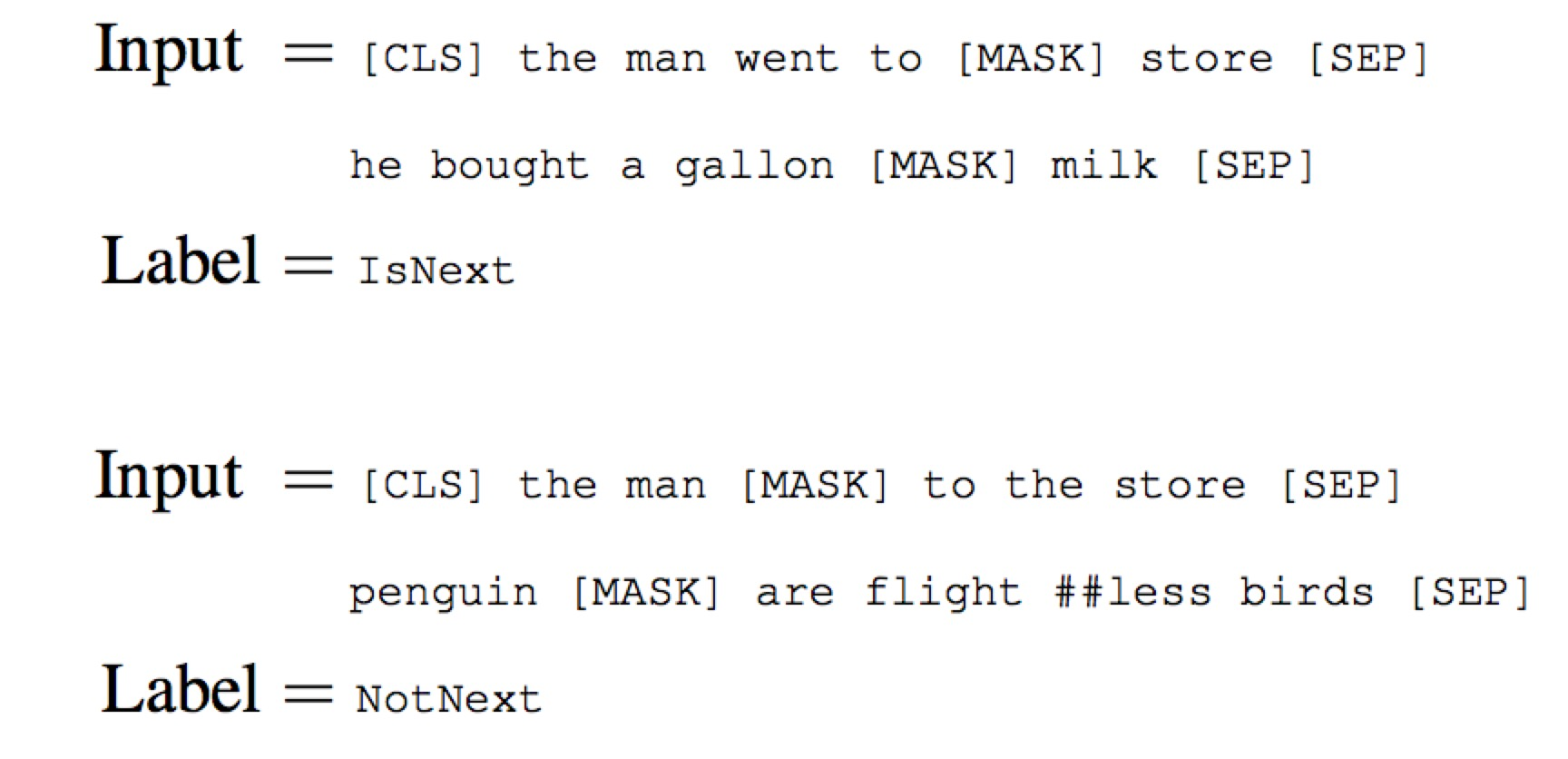具体的实现方式是两个连续的句子，开始和结束打上符号，两句之中打上分隔符，然后中一个二分类，反例的生成采用类似于word2vec的负采样。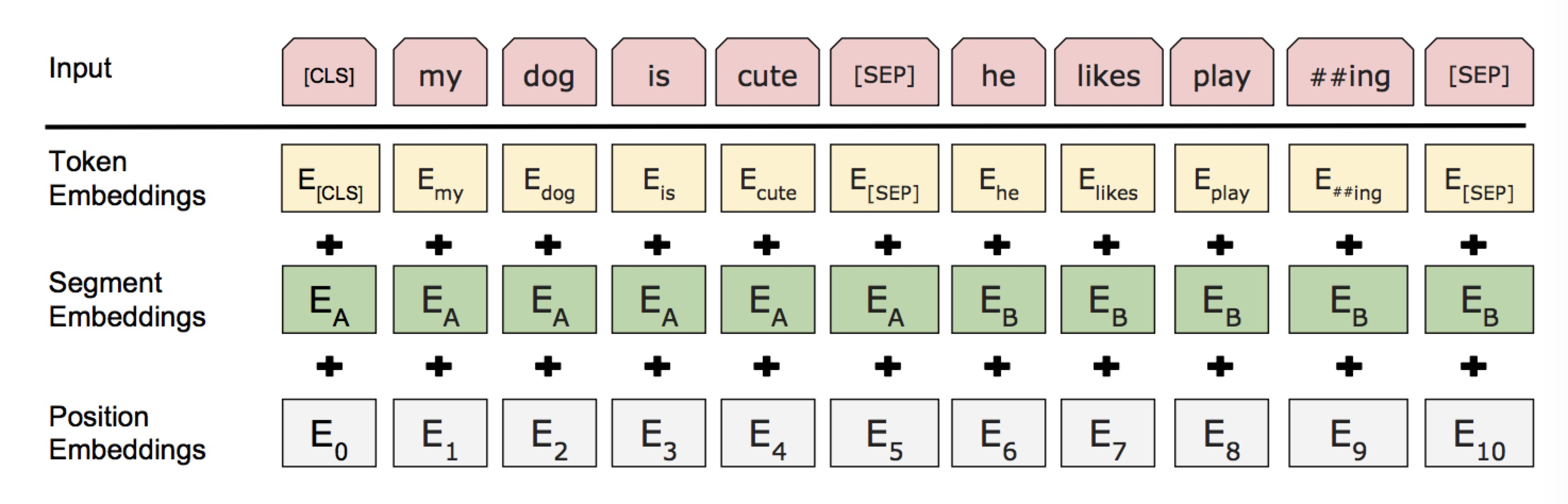这是整体的编码方式，Token Embedding是Transformer的embedding，加上segment embedding A和B，捕捉句子级别的特征，区分第一句和第二句，如果只有一个句子的情况就只有embedding A，加上每个位置赋予的Position embedding得到最终的embedding。

BERT的实验结果：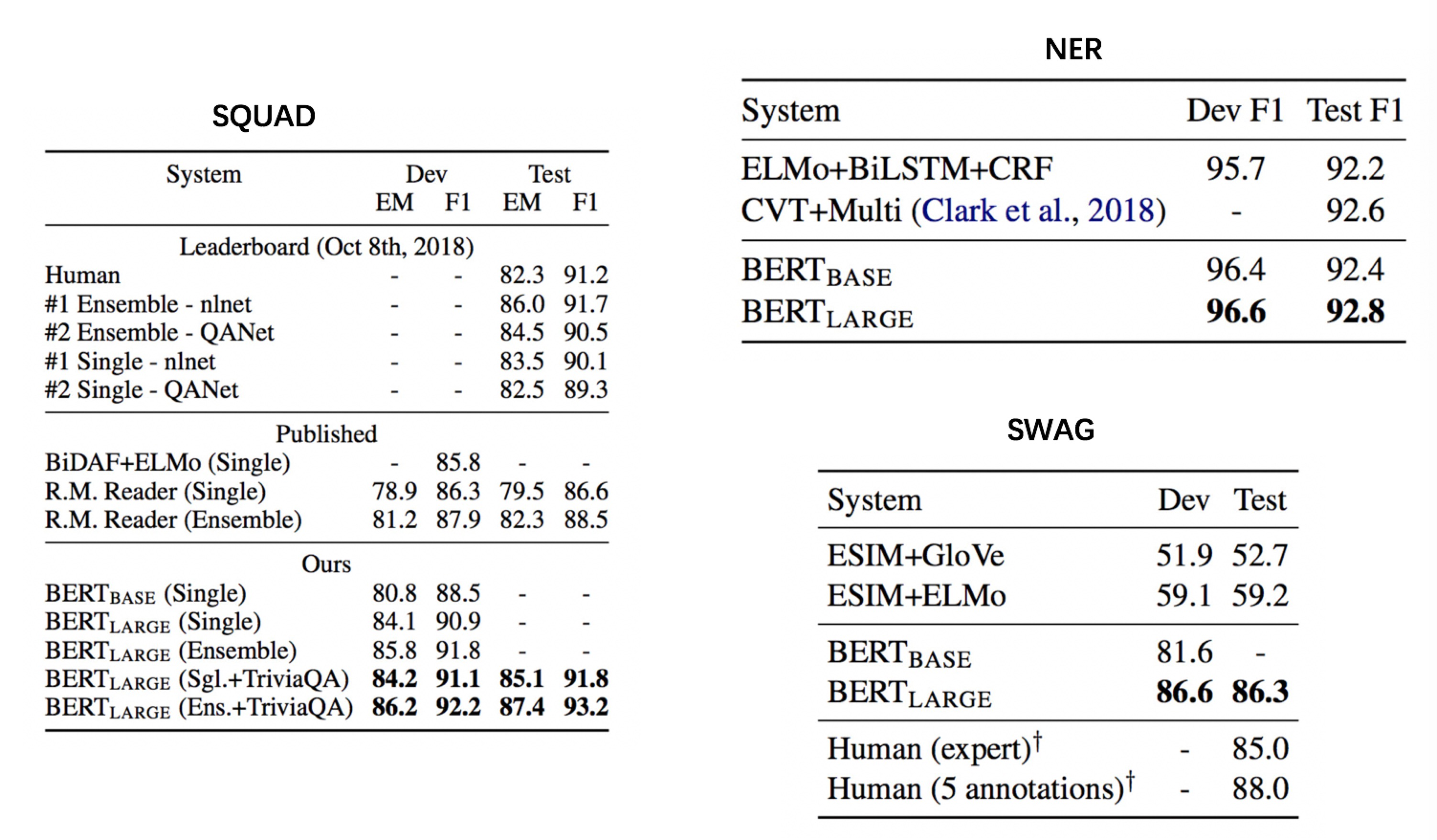参考文献：

1、word2vec：Efficient Estimation of Word Representation in Vector Space
2、Glove：Global vectors for word representation
3、ELMo：Deep contextualized word representations
4、Transformer：Attention is all you need
5、GPT：Improving Language Understanding by Generative Pre-Training
6、BERT： Pre-training of Deep Bidirectional Transformers for Language Understanding

展开全文• 主要给大家介绍了关于Three.js中矩阵和向量使用的相关资料，文中通过示例代码介绍的非常详细，对大家学习或者使用Three.js具有一定的参考学习价值，需要的朋友们下面来一起学习学习吧
• 以分类精度为目标的传统分类算法通常假定:每个样本的误分类具有同样的代价且每类样本数大致相等.但现实数据挖掘中该假定不成立时,这些算法的直接应用不能取得理想的分类和预测.针对此缺隙,并基于标准的SVM,通过在SVM...
• 双线性约束向量联合训练模型在实体消歧中的应用，陈辉，魏宝刚，综述文章：以背景、研究现状、研究用途的结构书写,篇幅以150～300字左右为宜,不用第一人称做主语,不与正文语句重复。一般研究性文章命名实体消歧 联合训练 词向量
• 支持向量机是机器学习领域里最强的几种分类器之一，被广泛的运用于各种分类回归问题，如果不考虑集成学习算法以及近几年出现的深度学习算法，支持向量机的性能可以说是在学习领域具有统治地位，在一些中小型的数据集...

支持向量机是机器学习领域里最强的几种分类器之一，被广泛的运用于各种分类回归问题，如果不考虑集成学习算法以及近几年出现的深度学习算法，支持向量机的性能可以说是在学习领域具有统治地位，在一些中小型的数据集上它的性能甚至能够超过一些深度学习网络。其基本原理相当简单，但是模型的求解和优化却十分复杂，很难描述清楚，这里我会一步一步，尽我所能分章节的将它总结完善

##模型篇
· 支持向量机：模型篇1–支持向量与间隔
· 支持向量机：模型篇2–支持向量的拉格朗日对偶
· 支持向量机：模型篇3–对偶问题的求解: SMO算法
· 支持向量机：模型篇4–核函数与非线性优化
· 支持向量机：模型篇5–向量机的软间隔拓展

##代码篇
· 支持向量机：代码篇1-基于CVXPT优化函数求解
· 支持向量机：代码篇2-基于SMO算法求解

支持向量的拉格朗日对偶

在模型篇1中，我们给出了支持向量机最朴素的模型，现在将它重新写出如下：

o b j { m i n w , b 1 2 ∣ ∣ w ∣ ∣ 2 s . t y i ( w T x + b ) > = 1 , i = 1 , 2 , . . . , m obj \left\{\begin{matrix} min_{\mathbf{w},b}\frac{1}{2}||\mathbf{w}||^{2}\\ s.t y_{i} (\mathbf{w^{T}}\mathbf{x}+b ) >=1 ,i = 1,2,...,m\end{matrix}\right.

当写出这个模型以后，我们可以发现这个目标函数是一个二次的，约束条件是线性的，所以它是一个凸的二次规划问题。对于这个问题，有很多的二次规划函数包可以解决，但是对于这个问题的求解我们往往是通过求解它的拉格朗日对偶问题来获得最优解，主要原因有以下两点

· 对偶问题可以得到更高效的求解
· 对偶问题可以方便的引入核函数，从而可以将支持向量机由线性推广到非线性

在开始模型的对偶转化之前，读者们需要了解什么是拉格朗日对偶，请花费10分钟了解我的另一篇博文之后再接着往下学习
人工智能里的数学修炼 | 约束问题的优化求解：拉格朗日乘子法、KKT条件与对偶问题

在了解了上面这篇博文之后，我们只需要根据文章里的套路来就可以了
首先将原模型通过拉格朗日乘子法,添加算子 a i > 0 a_{i}>0 记为

L ( w , a , b ) = 1 2 ∥ w ∥ 2 − ∑ i = 1 n a i ( y i ( w T x i + b ) − 1 ) L (w,a,b) = \frac{1}{2}\left \| w \right \|^{2} - \sum_{i = 1}^{n}a_{i}(y_{i}(w^{T}x_{i}+b)-1)

再写出该模型的极小极大形式
m i n w , b m a x a i ⩾ 0 L ( w , a , b ) = p ∗ min_{w,b}max_{a_{i}\geqslant0}L (w,a,b) = p^{*}

这里的 p ∗ p^{*} 表示的是这个问题的最优值，它与最初的问题是等价的。现在，我们将式子中的最小和最大的位置换一下，得到对偶问题
m a x a i ⩾ 0 m i n w , b L ( w , a , b ) = d ∗ max_{a_{i}\geqslant0}min_{w,b}L (w,a,b) = d^{*}

很容易理解的，我们有 p ∗ ⩾ d ∗ p^{*} \geqslant d^{*} ，即极大值的极小值大于极小值的极大值，这在上面那篇博文中有严格证明
现在我们的目的很明确，就是 通过求解对偶问题的极大值来求得原问题的极小值，但是 p ∗ ⩾ d ∗ p^{*} \geqslant d^{*} 即对偶的极大值和原问题的极小值不一定相等，是大于等于的关系，那么什么时候取到相等关系呢？显然的，那就是当原问题为凸优化问题并且满足KKT条件的时候！
本文在最后会给出该问题具体的KKT形式，现在，先让我们尝试将对偶问题转化为更易求解的形式

（1）首先固定住 a a 分别对 w , b w,b 求偏导，并令导数为0

∂ L ∂ w = 0 → w = ∑ i = 1 n a i y i x i \frac{\partial L}{\partial w} = 0 \rightarrow w = \sum_{i = 1}^{n}a_{i}y_{i}x_{i}
∂ L ∂ b = 0 → ∑ i = 1 n a i y i = 0 \frac{\partial L}{\partial b} = 0 \rightarrow \sum_{i = 1}^{n}a_{i}y_{i} = 0

(2) 将求导结果待回对偶式 L L ，可以得
L ( w , b , a ) = ∑ i = 1 n a i − 1 2 ∑ i , j = 1 n a i a j y i y j x i T x j L(w,b,a) = \sum_{i = 1}^{n}a_{i}- \frac{1}{2} \sum_{i,j = 1}^{n}a_{i}a_{j}y_{i}y_{j}x_{i}^{T}x_{j}

关于这一公式的具体推导过程是相当复杂的，如下图所示：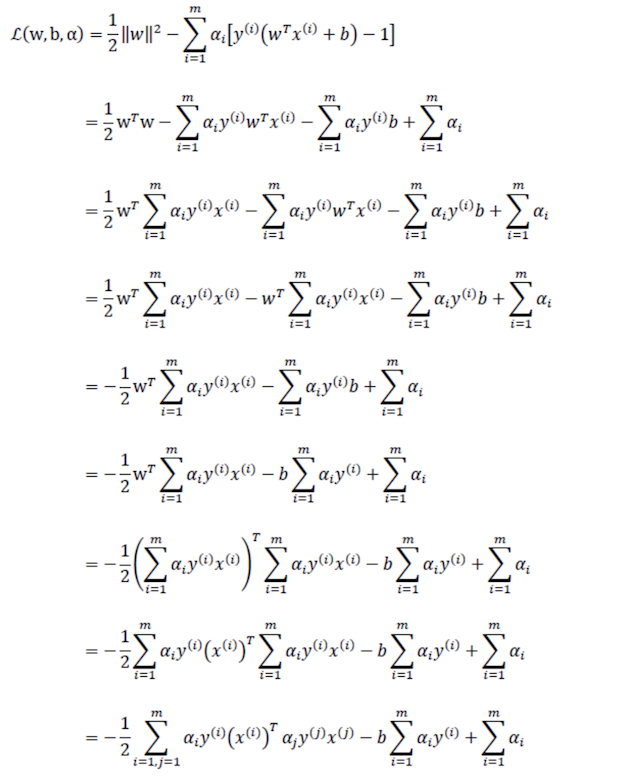最后，就可以得到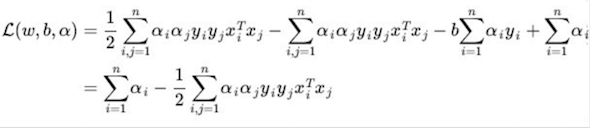（3）经过带入化简之后，得到的式子里已经没有了 w , b w,b ，现在的问题是变化 a i a_{i} 以求式子的极大值。在我们求解出 a i a_{i} 后是可以通过上面求导的式子重新解出 w , b w,b 得到我们需要的超平面 f ( x ) f(x) ，即

w = ∑ i = 1 n a i y i x i w = \sum_{i = 1}^{n}a_{i}y_{i}x_{i}
b = − m a x i , y i = − 1 w T x i + m i n i , y i = 1 w T x i 2 b = - \frac{max_{i,y_{i}= -1}w^{T}x_{i}+min_{i,y_{i}= 1}w^{T}x_{i}}{2}

f ( x ) = w T x + b f(x) = w^{T}x+b

(4) 最后，我们可以给出我们化简后的对偶优化目标以及它的相关约束
m a x a ∑ i = 1 n a i − 1 2 ∑ i , j = 1 n a i a j y i y j x i T x j max_{a} \sum_{i = 1}^{n}a_{i}- \frac{1}{2} \sum_{i,j = 1}^{n}a_{i}a_{j}y_{i}y_{j}x_{i}^{T}x_{j}
s . t . a i > = 0 , i = 1 , . . . , n s.t. a_{i} >= 0,i= 1,...,n
∑ i = 1 n a i y i = 0 \sum_{i = 1}^{n}a_{i}y_{i} = 0

上式应该满足KKT条件的约束，KKT条件为
{ a i > = 0 y i f ( x i ) − 1 > = 0 a i ( y i f ( x i ) − 1 ) = 0 \left\{\begin{matrix}a_{i} >=0\\y_{i}f(x_{i})-1 >= 0 \\ a_{i}(y_{i}f(x_{i})-1) = 0\end{matrix}\right.

这样，我们就完成了从支持向量机的原始模型到对偶模型以及对偶模型化简的全部推导。这里要提及一个点是，从上述的KKT条件中，我们可以看出，对于任意的一个样本 ( x i , y i ) (x_{i},y_{i}) ，总有 a i = 0 a_{i} = 0 y i f ( x i ) = 1 y_{i}f(x_{i}) = 1 。即， a i = 0 a_{i} = 0 ，则该样本不会出现在超平面 $f(x)$ 的参数 w w 的计算中，不会对超平面的计算产生任何影响；若 a i > 0 a_{i} > 0 ，则必有 y i f ( x i ) = 1 y_{i}f(x_{i}) = 1 ，所以该样本点位于最大间隔边界上，是一个支持向量。这显示出支持向量机的一个充要性质： 训练完成后，大部分的训练样本都不需要保留，最终模型仅与支持向量有关

拉格朗日对偶的重要意义在于将对 w w 的计算提前并消除了 w w ,将优化函数变为了关于拉格朗日乘子的二次规划问题。关于对偶优化目标的求解我们将会在下一篇博文中给出解答

更多资源下载

微信搜索“老和山算法指南”获取更多下载链接与技术交流群有问题可以私信博主，点赞关注的一般都会回复，一起努力，谢谢支持。

展开全文机器学习 支持向量机 对偶问题 KKT条件
• 最近在学习用cocos creator开发微信小程序，在入门案例中，需要计算两点之间的向量及其长。 教学视频中使用的是cocos以前的版本，老师使用： cc.pSub(this.startposition , this.endposition) 便轻松取得了两点...微信小游戏 JavaScript
• 在cs224n Lecture 3: GloVe skipgram cbow lsa 等方法对比 / 词向量评估 /超参数调节 总结 中， 我们知道，以往生成词向量有两种算法，一种是基于矩阵分解（Matrix Factorization）的，代表有LSA，改进的HAL算法等...GloVe NLP
• Word2Vec模型计算词向量

千次阅读 2018-06-24 15:44:28
简单来说就是通过训练大量文本，将文本中的词用向量形式表示，这个向量我们称之为词向量，转换成词向量的好处在于，我们可以通过计算两个词的词向量之间的距离，从而得知两个词之间的联系。比如“公交车”与“巴士”...
• 向量模板

2015-12-04 22:24:36
#include struct node{ double dis(node);//两点距离 //向量操作 node add(node);//加 double mul(node);//乘 node mul(double);//倍 double abs();//长 node unt();//单位化 node neg();//取
• 向量模板类

千次阅读 2018-01-01 23:00:52
数据结构之向量1.简述数据结构是数据项的结构化集合，其结构性表现为数据项之间的相互联系及作用，也可以理解为定义数据项之间的某种逻辑次序。而根据这种逻辑次序的复杂程度，大致可以将各种逻辑结构大致分为三大类...
• 阅读的第一篇词向量相关的文献是Tomas Mikolov 2013年的论文，其中提到了Bengio在2003的经典文章。经过一番努力，粗略的学习了这两篇文献，并查阅了相关的资料，现简单整理如下： 1、词向量 作为NLP的初学者，遇到...nlp
• 针对组合预测过程中的多误差统计向量的优劣评价问题，提出了一种优于个量相同条件下的多维度评价向量的排序模型，设定优劣排序的相关规则，根据规则给出整体优于函数，并对于优于个量相等优于程度不同的情况给出了...
• 本文介绍了向量的定义、向量、负向量、单位向量、零向量以及向量加减法的三种实现方法。人工智能 数学 线性代数 向量
• 如果一个向量不是单位向量，可以通过单位化将其转化为单位向量，即 非零向量除以向量，如下图所示 向量点乘 点乘只能发生在两个向量之间，且点乘时，两向量必须是单位向量，如果不是，需要将向量进行单位化后...OpenGL 向量 矩阵
• R语言学习——向量

千次阅读 2017-11-16 19:56:50
判断向量是否相等 > x > y (1,3,4) > all(x==y)  FALSE > identical(x,y)  FALSE 注意1:3是整数，c(1,2,3)是浮点数，它们不相等 ： > identical(1:3, c(1,2,3))  ...R语言 数据
• 已知向量A=(a,b),与它垂直向量B=k（b，-a），其中k为任意实数。 用于图纸中的尺寸标注。
• 使用BERT模型生成token级向量

千次阅读 2019-09-23 14:23:17
相信网上有很多方法可以生成BERT向量，最有代表性的一个就是bert as service，用几行代码就可以生成向量，但是这样生成的是句向量，也就是说，正确的做法是输入一句句子： 我是一个中国人，我热爱着中国的每一个...
• 向量指具有大小和方向的量，一般记做：a ，，，同时...向量的大小，也就是向量的长度（一般称作为 ），向量a的记为：，若，则 单位向量：即为1的向量向量：即为0的向量，零向量的方向是任意的 ...向量 向量的运算
• 本节主要介绍文本分类中的一种算法即向量空间模型，这个算法很经典，包含文本预处理、特征选择、特征权值计算、分类算法、这是VSM的几个主要步骤，在宗老师的书里都有详细的讲解，这里也会进行深入的讲解，浅显易懂...
• NLP中的词向量及其应用

千次阅读 2019-01-15 08:07:59
向量基本上是一种单词表示形式，它将人类对语言的理解与机器的理解连接起来。词向量是文本在n维空间中的分布式表示。这些是解决大多数NLP问题所必需的。 领域适应是一种技术，它允许机器学习和转移学习模型来映射...NLP 词向量
• 向量的夹角就是向量两条向量所成角 其范围是在0到180度 而向量夹角的余弦值等于= 向量的乘积/向量模的积 即cos=ab/ (|a|·|b|)两向量夹角怎么求？？？给的是坐标，要求步骤详细点，多谢夹角为α=arccos...两个向量的夹角公式
• 向量加减法，向量的点积（乘），向量的叉积（乘） 向量 是用来表示既有大小又有方向的量，不过向量在空间中没有具体的位置，通常用一个加粗的小写字母来表示一个向量，或者不加粗顶上带有小箭头的小写字母来表示 ...计算机图形学 向量 向量点积 向量叉积
• 什么是向量 　在数学中，向量（也称为欧几里得向量、几何向量、矢量），指具有大小（magnitude）和方向的量。它可以形象化地表示为带箭头的线段。箭头所指：代表向量的方向；线段长度：代表向量的大小。与向量...线性代数 向量
• 《数学》本就是我们原本掌握却又遗忘的知识 一、知识纲要 1.向量的相关概念 （1）向量： 既有大小又又方向的量叫做向量，记为 AB→\overrightarrow{AB}AB 或者 a→\overrightarrow{a}a。...（2）向量向量的.
• http://en.wikibooks.org/wiki/GLSL_Programming/Applying_Matrix_Transformations
• 向量平行公式和垂直公式

千次阅读 2020-12-19 23:10:52
平面向量平行对应坐标交叉相乘相等，即x1y2=x2y，垂直是内积为0。方向相同或相反的非零向量叫做平行(或共线)向量.向量a、b平行(共线)，记作a∥b。零向量长度为零，是起点与终点重合的向量，其...两直线平行交叉相乘...